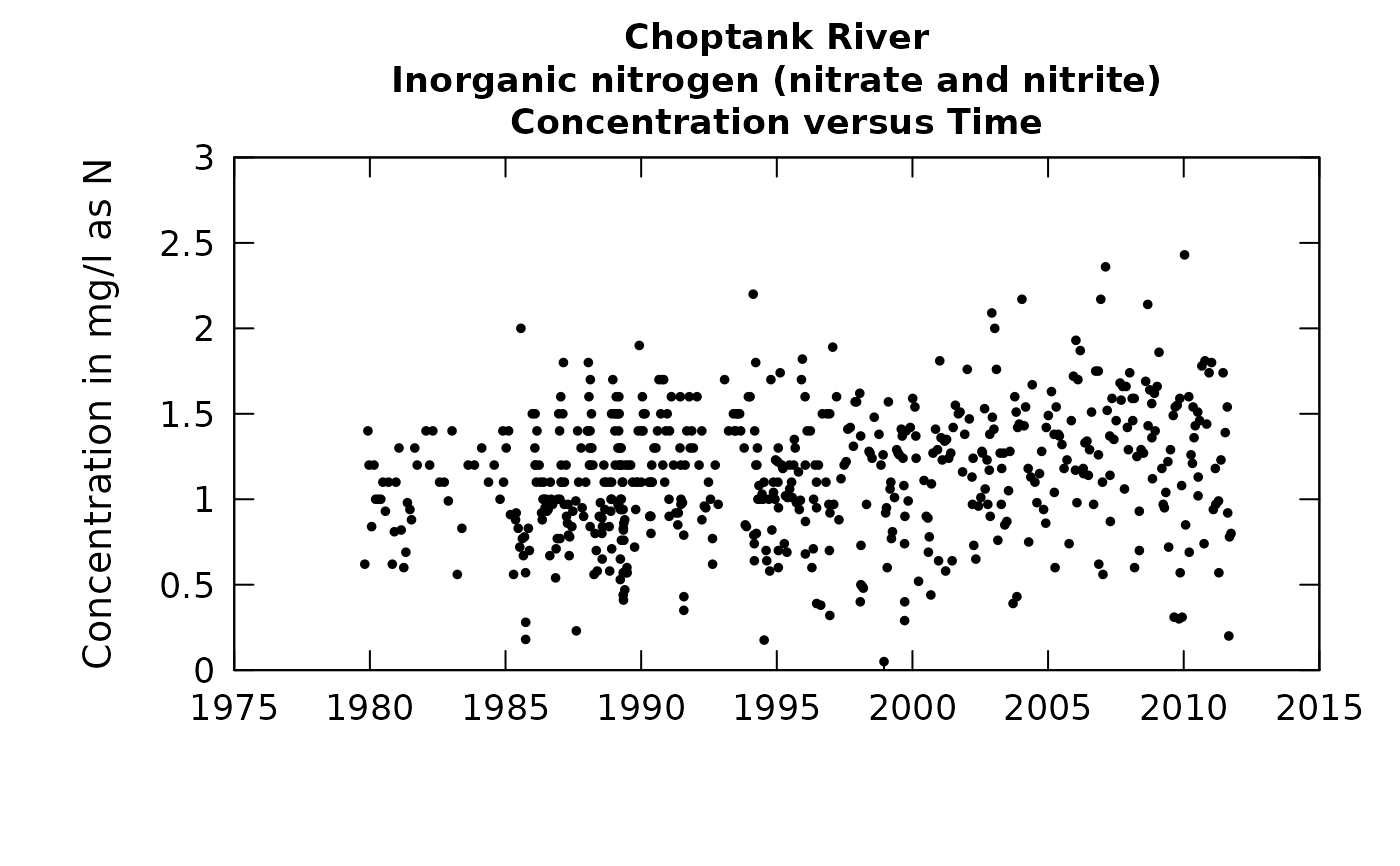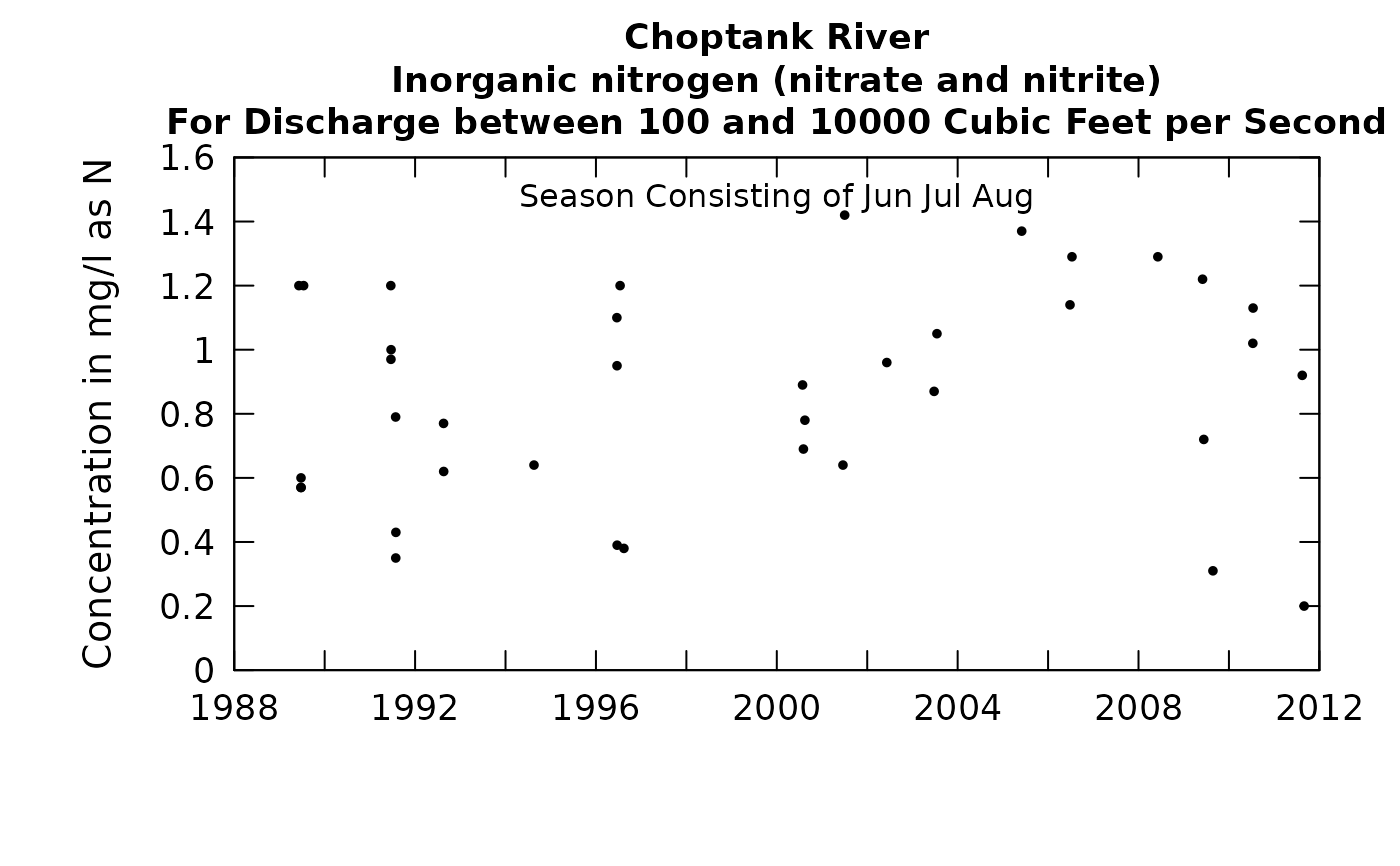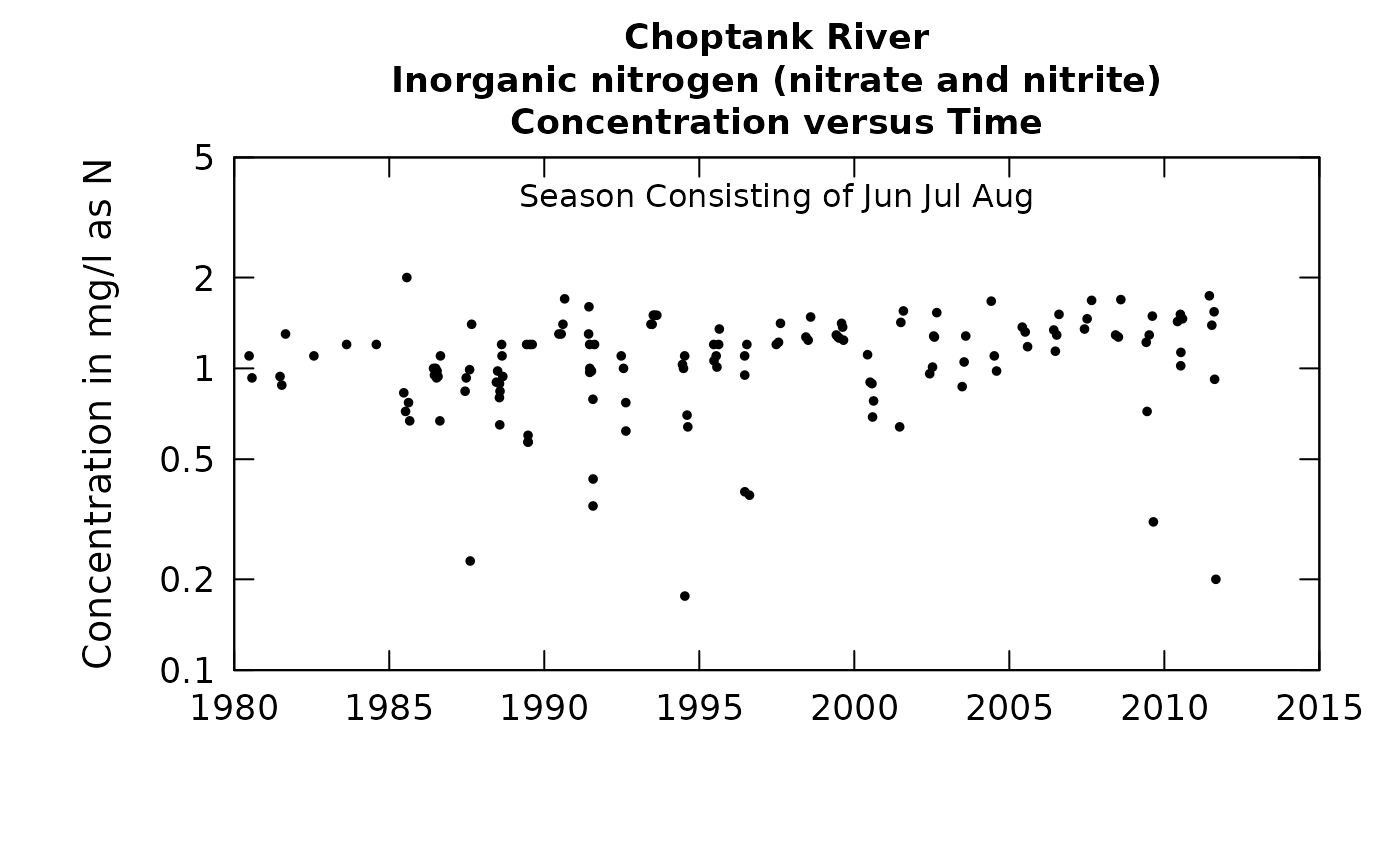This function allows the user to plot all of the data, but also to limit it in two ways. The data can be limited to only those observed concentrations collected in a specified discharge range. The data can also be limited to only those observed in certain months of the year. These two selection criteria can be combined. For example, we may only want to plot data for discharges between 100 and 500 cubic feet per second in the months of March, April and May.

Although there are a lot of optional arguments to this function, most are set to a logical default.

Data come from named list, which contains a Sample dataframe with the sample data, and an INFO dataframe with metadata.

## Usage

plotConcTime(eList, qUnit = 2, yearStart = NA, yearEnd = NA,
qLower = NA, qUpper = NA, randomCensored = FALSE, tinyPlot = FALSE,
concMax = NA, concMin = NA, printTitle = TRUE, logScale = FALSE,
cex = 0.8, cex.axis = 1.1, cex.main = 1.1, customPar = FALSE,
col = "black", lwd = 1, concLab = 1, usgsStyle = FALSE, ...)

## Arguments

eList

named list with at least the Sample and INFO dataframes

qUnit

object of qUnit class printqUnitCheatSheet, or numeric represented the short code, or character representing the descriptive name.

yearStart

numeric is the calendar year containing the first estimated annual value to be plotted, default is NA (which allows it to be set automatically by the data)

yearEnd

numeric is the calendar year just after the last estimated annual value to be plotted, default is NA (which allows it to be set automatically by the data)

qLower

numeric the lower bound on values of discharge used to select the data points to be plotted, units are those specified by qUnit, default = NA which is equivalent to a lower bound of zero but if the desired lower bound is zero use qLower = NA

qUpper

numeric the upper bound on values of discharge for selection of data points to be plotted, units are those specified by qUnit, default = NA which is equivalent to an upper bound of infinity

randomCensored

logical. Show censored values as randomized.

tinyPlot

logical variable, if TRUE plot is designed to be plotted small as part of a multipart figure, default is FALSE.

concMax

numeric value for the maximum value to be used on the vertical axis, default is NA (which allows it to be set automatically by the data)

concMin

numeric value for lower limit on concentration shown on the vertical log graph, default is NA (which causes the lower limit to be set automatically, based on the data). This value is ignored for linear scales, using 0 as the minimum value for the concentration axis.

printTitle

logical variable if TRUE title is printed, if FALSE title is not printed (this is best for a multi-plot figure).

logScale

logical. If TRUE concentration is plotted on a log axis, default FALSE.

cex

numerical value giving the amount by which plotting symbols should be magnified.

cex.axis

magnification to be used for axis annotation relative to the current setting of cex.

cex.main

magnification to be used for main titles relative to the current setting of cex.

customPar

logical defaults to FALSE. If TRUE, par() should be set by user before calling this function. (for example, adjusting margins with par(mar=c(5,5,5,5))). If customPar FALSE, EGRET chooses the best margins depending on tinyPlot.

col

color of points on plot, see ?par 'Color Specification'

lwd

number line width.

concLab

object of concUnit class, or numeric represented the short code, or character representing the descriptive name. By default, this argument sets concentration labels to use either Concentration or Conc (for tiny plots). Units are taken from the eList$INFO$param.units. To use any other words than "Concentration" see vignette(topic = "units", package = "EGRET").

usgsStyle

logical option to use USGS style guidelines. Setting this option to TRUE does NOT guarantee USGS compliance. It will only change automatically generated labels

...

arbitrary functions sent to the generic plotting function. See ?par for details on possible parameters.

## Details

The function has two possible ways to plot censored values (e.g. "less-than values").

The default is to plot them as a vertical line that goes from the reporting limit down to the bottom of the graph.

The alternative is to set randomCensored = TRUE. In this case a random value is used for plotting the individual sample value. This random value lies between the reporting limit and zero and it is distributed as a truncated log normal based on the fitted WRTDS model.

The function makeAugmentedSample must be run first if randomCensored = TRUE. Running makeAugmentedSample requires that modelEstimation has already been run.

These random censored values are used to create more readable plots and are not used in any computations about the data set. The random censored values are shown as open circles and the non-censored data are shown as filled dots.

selectDays, genericEGRETDotPlot

## Examples

eList <- Choptank_eList
# Water year:
plotConcTime(eList)# Graphs consisting of Jun-Aug
eList <- setPA(eList, paStart=6,paLong=3)
plotConcTime(eList,
qUnit = 1,
qLower = 100,
qUpper = 10000)plotConcTime(eList, logScale=TRUE)plotConcTime(eList,
qUnit = 1,
qLower = 100, qUpper = 10000,
randomCensored = TRUE)